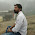# Geeksforgeeks Solution For " Find number of days between two given dates "

GeeksforGeeks Solution For School Domain .Below You Can Find The Solution Of Basic ,Easy ,Medium ,Hard .You Can Also Direct Submit Your Solution to Geeksforgeeks Same Problem .You Need to login then you can submit you answers

Problem :- Given two dates, find total number of days between them. The count of days must be calculated in O(1) time and O(1) auxiliary space.

Note :- The system follows Gregorian calendar from the beginning of the time.

Solution :-

#include<bits/stdc++.h>
using namespace std;

struct Date
{
int d, m, y;
};
const int monthDays = {31, 28, 31, 30, 31, 30,31, 31, 30, 31, 30, 31};

int countLeapYears(Date d)
{
int years = d.y;
if (d.m <= 2)
years--;
return years / 4 - years / 100 + years / 400;
}
int getDifference(Date dt1, Date dt2)
{
long int n1 = dt1.y*365 + dt1.d;
for (int i=0; i<dt1.m - 1; i++)
n1 += monthDays[i];
n1 += countLeapYears(dt1);

long int n2 = dt2.y*365 + dt2.d;
for (int i=0; i<dt2.m - 1; i++)
n2 += monthDays[i];
n2 += countLeapYears(dt2);
return (n2 - n1);
}

int main()
{
int t,d,m,y;
cin>>t;
while(t--)
{
Date dt1,dt2;
cin>>d>>m>>y;
dt1={d,m,y};
cin>>d>>m>>y;
dt2={d,m,y};
cout<<abs(getDifference(dt1, dt2))<<endl;
}
return 0;
}

Output:-

1.1.